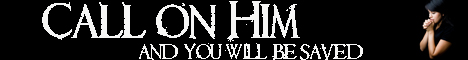Note:  Do not rely on this information. It is very old.

# Total Reflection

Total Reflection. When a ray of light passes from one medium, M, into a denser medium, M', it is bent in towards the normal to the dividing surface. [REFRACTION.] The ray is naturally bent away from the normal when it passes from M' to M. The incident ray, I O, will therefore be bent into the position O R. As the angle, I O N, is increased, O R makes a smaller and smaller angle with the surface, until when the incident ray is in a certain position, I' O, the refracted ray is in the surface (O R'). Upon inclining I' O still more to the normal we fail to get any refracted ray at all - all the light obeys the laws of reflection - and it is said to be totally reflected. If M be air and u the index of refraction from air to M', then 1/u is the index of refraction from M' to air. Therefore, 1/u = sin I O N / sin R O N' and total reflection begins when R O N has become R' O N', i.e. 90°. Hence, 1/u = sin I' O N. This angle is called the critical angle. Hence, the sine of the critical angle is the reciprocal of the index of refraction of the medium, and total reflection occurs when incident rays from the denser medium meet the surface at any angle greater than this critical angle.